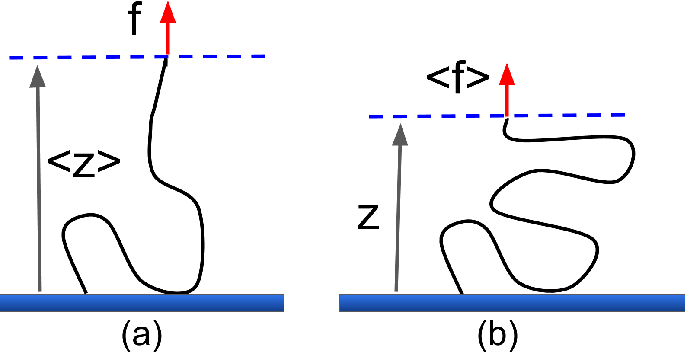耶鲁基础物理 6.5 保守力- 阅读剩余部分 -

耶鲁基础物理5.4摩擦与动能定理- 阅读剩余部分 -

质点沿粗糙球面滑下的命运- 阅读剩余部分 -

Mathematica 画受力示意图

 1 2 3 4 5 6 7 8 9 10 11 12 13 14 15 16 17 18
 g1 = Graphics[Line[{{0, 0}, {20, 0}}]]; g2 = Graphics[Line[{{0, 0}, {15, 15}}]]; g3 = Graphics[{Opacity[0.2], Blue, Rotate[Rectangle[{8, 8}, {12, 12}], 45 Degree, {Left, Bottom}]}]; g4 = Graphics[{Blue, Thickness[0.01], Arrow[{{8, 10.8}, {8, 2.8}}]}]; g5 = Graphics[{Cyan, Thickness[0.01], Arrow[{{8, 10.8}, {3.8, 15}}]}]; g6 = Graphics[{Orange, Thickness[0.01], Arrow[{{2.4, 5.2}, {6.6, 9.4}}]}]; g7 = Graphics[ Text[StyleForm["重力", FontSize -> 14, FontWeight -> "Bold"], {9, 6.8}, {0, 1}, {0, -1}]]; g8 = Graphics[ Text[StyleForm["支持力", FontSize -> 14, FontWeight -> "Bold"], {6, 13}, {0, -1}, {1, -1}]]; g9 = Graphics[ Text[StyleForm["摩擦力", FontSize -> 14, FontWeight -> "Bold"], {4.5, 7.2}, {0, 1}, {1, 1}]]; Show[g1, g2, g3, g4, g5, g6, g7, g8, g9]接枝高斯链系综不等价性- 阅读剩余部分 -